# Newtons First Law Newtons Second Law Newtons Third

• Slides: 51Newton’s First Law Newton’s Second Law Newton’s Third Law 1 pt 1 pt 2 pt 2 pt 3 pt 3 pt 4 pt 4 pt 5 pt 5 pt Kinematics MeasurementThe resistance of an object to a change in velocityWhat is inertia?What a baseball does if you throw it in spaceWhat is continue moving in a straight line?Why things thrown on Earth eventually stopWhat is friction?The quantity which determines an object’s inertiaWhat is the mass?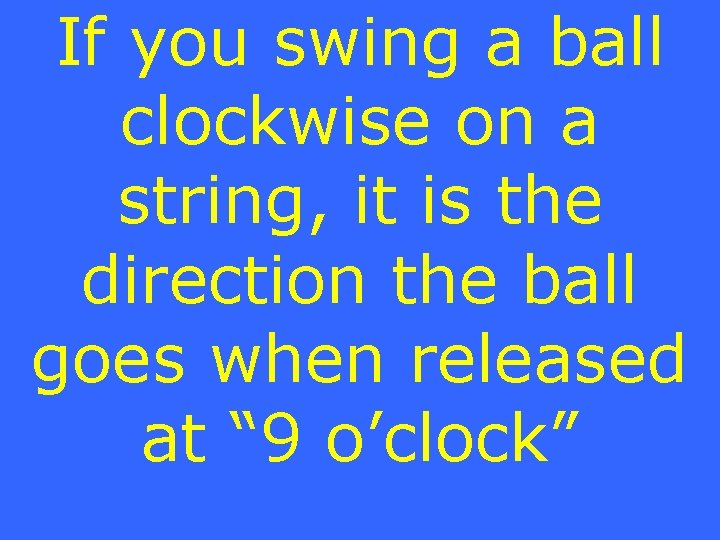If you swing a ball clockwise on a string, it is the direction the ball goes when released at “ 9 o’clock”What is upwards?It is the cause of an object’s accelerationWhat is net force?Acceleration is inversely proportional to itWhat is the mass?The force with which gravity pulls on a 10 kg objectWhat is 100 N?The acceleration of a 50 kg object pulled with 10 N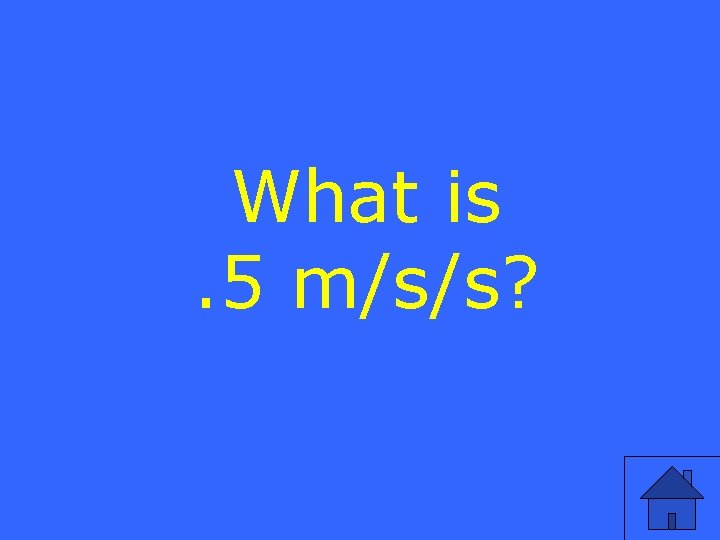What is. 5 m/s/s?The time during which a net force acting on an object whose graph is:What is during the first second?If the action force is you pulling on a rope, this is the reaction forceWhat is the rope pulling on you?The amount of force with which a scale pushes up on my feet (if I am still)What is my weight?It pushes on airplanes to make them goWhat is air?If an action force is Object A pushing Object B, then the reaction force acts on what objectWhat is object A?The force of a strongman pulling on you plus the force of you pulling on the strongmanWhat is 0 N?Velocity equals (final position initial position) divided by thisWhat is the change in time?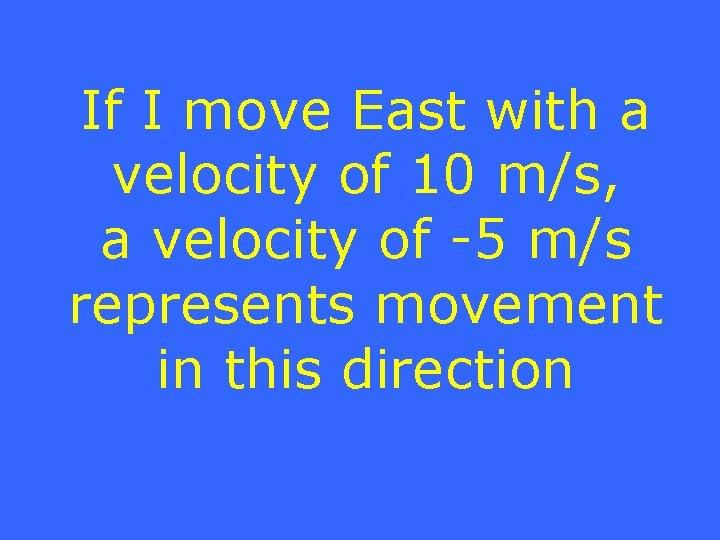If I move East with a velocity of 10 m/s, a velocity of -5 m/s represents movement in this directionWhat is West?It is defined as a change in velocity divided by a change in timeWhat is acceleration?The equation 2 d=1/2 at , solved for acceleration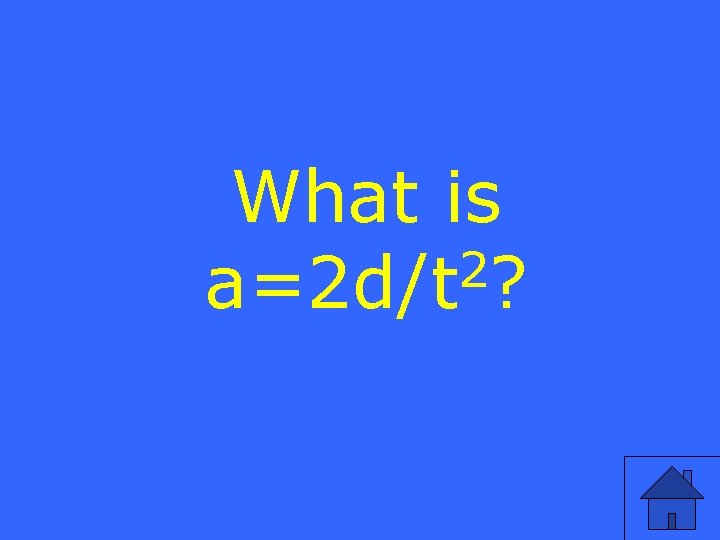What is 2 a=2 d/t ?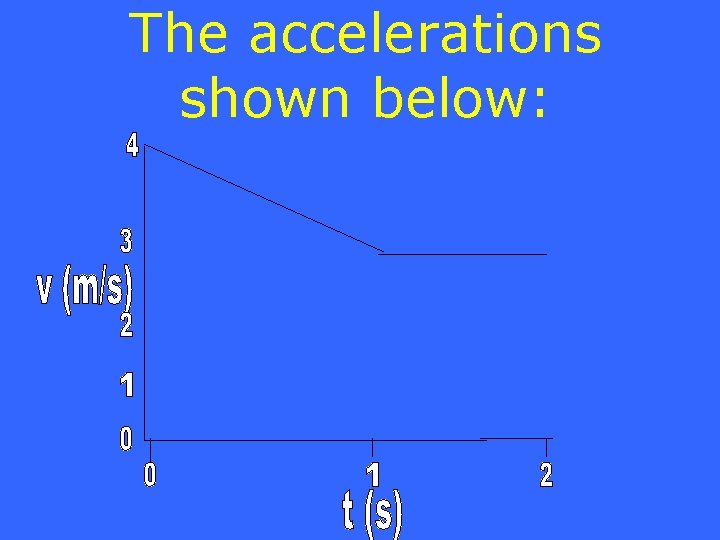The accelerations shown below: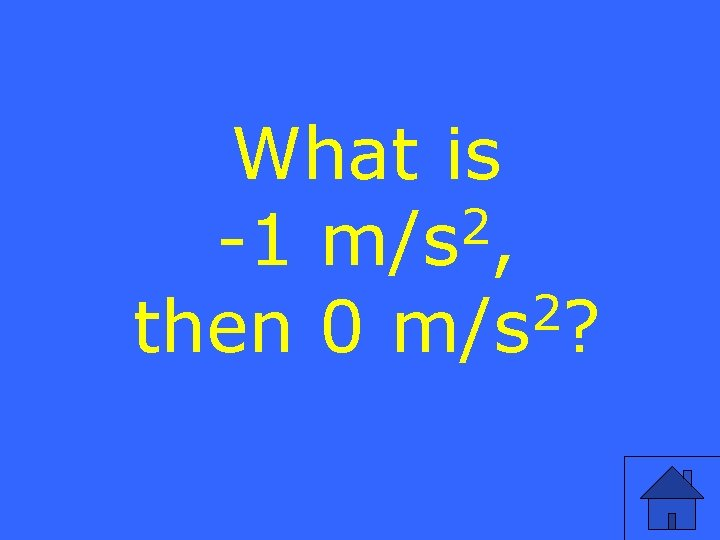What is 2 -1 m/s , 2 then 0 m/s ?It is the standard (SI) unit of forceWhat is the Newton (N)?The difference between your measurement and the true valueWhat is error?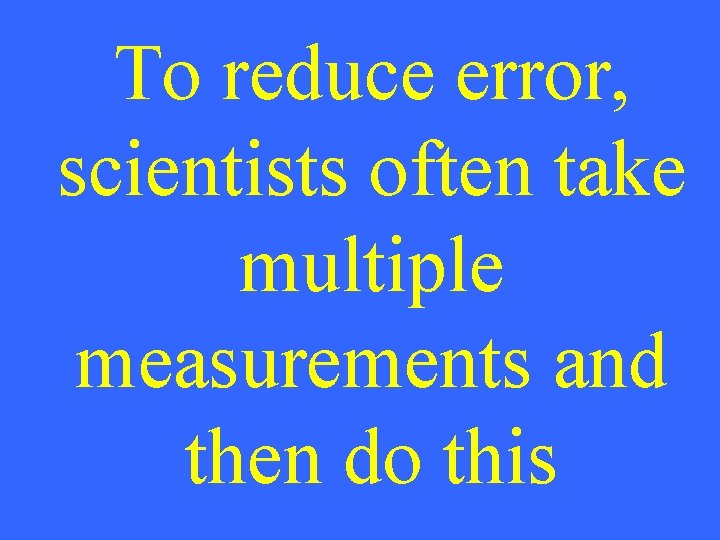To reduce error, scientists often take multiple measurements and then do thisWhat is take an average?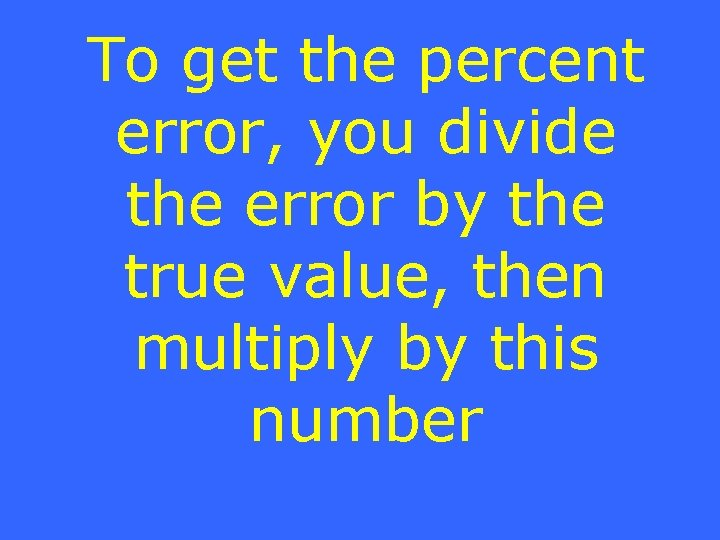To get the percent error, you divide the error by the true value, then multiply by this numberWhat is 100?If your measurements are consistently wrong in one direction, it might be due to this type of errorWhat is “Systematic error”?